### Barium Nitrate

This form shows a crystal of Barium Nitrate exhibiting isometric tetartohedral crystallization.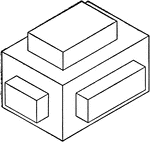### Isometric of a Block

Isometric of a wooden block.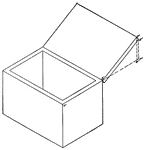### Isometric of a Box With a Cover

Isometric of a box with a cover.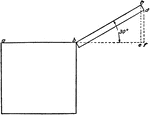### Isometric of a Box With a Cover

Isometric of a box with a cover - end view.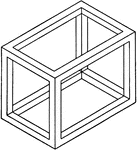### Isometric Of A Box

Illustration of the isometric of a skeleton of a box.### Isometric of a Brace

Isometric of a wooden brace.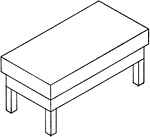### Isometric Of A Carpenter's Bench

Illustration of the isometric of a carpenter's bench.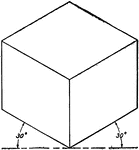### Isometric of a Cube

Isometric of a cube with 30°.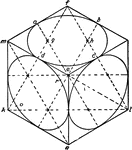### Isometric of a Cube With Circles Inscribed

Isometric of a cube with circles inscribed on its faces.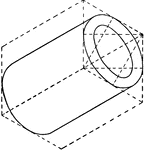### Hollow Cylinder

Illustration of the isometric of a hollow cylinder.### Hollow Cylinder

Illustration of a shaded section of a hollow cylinder viewed from the side.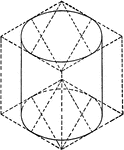### Isometric of a Cylinder

Isometric of a cylinder.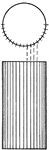### Side And Top Views Of A Cylinder

Illustration of the plan and shaded elevation of a cylinder. The cylinder is viewed from the side and…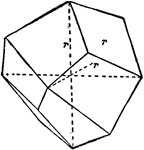### Deltohedron

Principal forms of the isometric system: deltohedron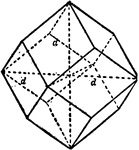### Dodecahedron

Principal forms of the isometric system: dodecahedron.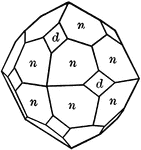### Garnet

"Isometric. Common forms dodecahedron amd trapezohedron, often in combination." — Ford, 1912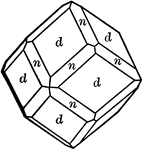### Garnet

"Isometric. Common forms dodecahedron amd trapezohedron, often in combination." — Ford, 1912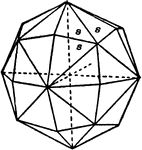### Hexoctahedron

Principal forms of the isometric system: hexoctahedron.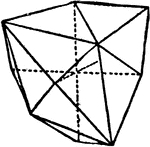### Hextetrahedron

Principal forms of the isometric system: hextetrahedron.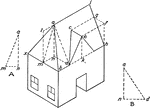### Isometric of a House

Isometric outline of a house.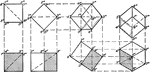### Development of an Isometric of a Cube

Development of an isometric of a cube.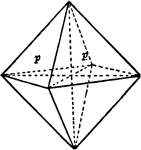### Octahedron

Principal forms of the isometric system: octahedron.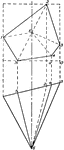### Isometric of Oblique Pentagonal Pyramid

Illustration of the isometric of an oblique pentagonal pyramid.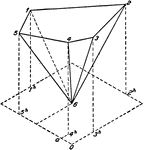### Oblique Pentagonal Pyramid

Illustration of a plan and elevation of an oblique pentagonal pyramid.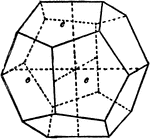### Pyritohedron

Principal forms of the isometric system: pyritohedron.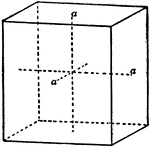### Rectangular Prism

Principal forms of the isometric system: rectangular prism.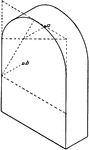### Prism With Semicircular Top

Isometric of a prism with a semicircular top.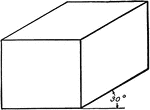### Oblique View Of Rectangular Solid

Illustration of an oblique view of a rectangular solid/prism at 30°.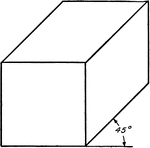### Oblique View Of Rectangular Solid

Illustration of an oblique view of a rectangular solid/prism at 45°.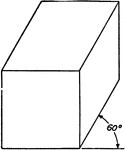### Oblique View Of Rectangular Solid

Illustration of an oblique view of a rectangular solid/prism at 60°.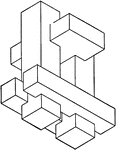### Combination of Geometric Solids

Illustration of model created by combining non regular geometric solids.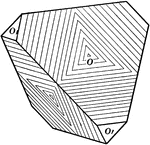### Sphalerite

"Isometric; tetrahedral. Tetrahedron form." — Ford, 1912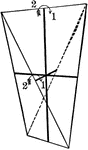### Symmetry of Sphenoidal class

"The Sphenoidal Class corresponds in the Tetragonal system to the Tetrahedral class in the Isometric…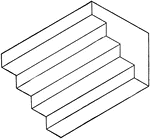### Isometric of Stairs

Illustration of stairs; 3-dimensional view of 4 steps. The staircase can be created by combining/stacking…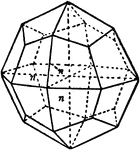### Tetragonal Trisoctahedron

Principal forms of the isometric system: tetragonal trisoctahedron.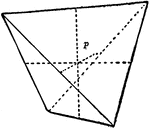### Tetrahedron

Principal forms of the isometric system: tetrahedron.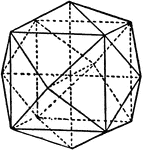### Tetrahexahedron

Principal forms of the isometric system: tetrahexahedron.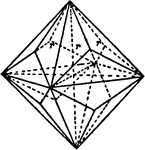### Trigonal Trisoctahedron

Principal forms of the isometric system: trigonal trisoctahedron.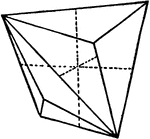### Tristetrahedron

Principal forms of the isometric system: tristetrahedron.